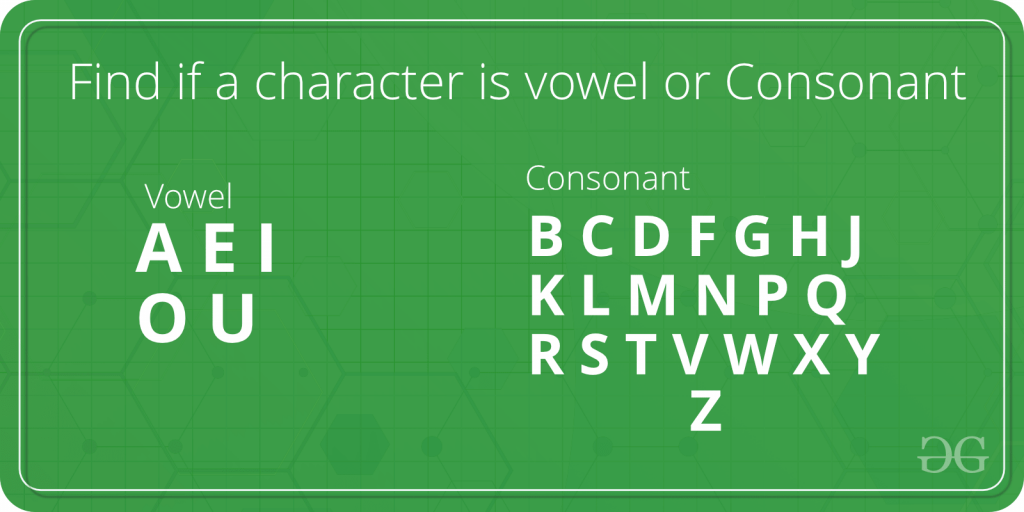# Java Program to Check Whether the Character is Vowel or Consonant

For any given character, we need to check if it is a vowel or a consonant. As we know, vowels are ‘a’, ‘e’, ‘i’, ‘o’, ‘u’ and all the other characters (i.e. ‘b’, ‘c’, ‘d’, ‘f’ …..) are consonants.Examples:

```Input : char = 'r'
Output : Consonant

Input : char = 'e'
Output : Vowel```

Here, in the below implementation we will check if the stated character corresponds to any of the five vowels. And if it matches, “Vowel” is printed, else “Consonant” is printed.

Example 1:

## Java

 `// java program to check whether input ` `// character is a vowel or consonant ` ` `  `import` `java.io.*; ` ` `  `public` `class` `geek { ` ` `  `    ``// Function to find whether an input ` `    ``// character is vowel or not ` `    ``static` `void` `Vowel_Or_Consonant(``char` `y) ` `    ``{ ` `        ``if` `(y == ``'a'` `|| y == ``'e'` `|| y == ``'i'` `|| y == ``'o'` `            ``|| y == ``'u'``) ` `            ``System.out.println(``"It is a Vowel."``); ` `        ``else` `            ``System.out.println(``"It is a Consonant."``); ` `    ``} ` ` `  `    ``// The Driver code ` `    ``static` `public` `void` `main(String[] args) ` `    ``{ ` `        ``Vowel_Or_Consonant(``'b'``); ` `        ``Vowel_Or_Consonant(``'u'``); ` `    ``} ` `}`

Output

```It is a Consonant.
It is a Vowel.```

Example 2:

• Alteration for capital letters.

## Java

 `// java program to check whether input ` `// character is a vowel or consonant ` ` `  `import` `java.io.*; ` ` `  `public` `class` `geek { ` ` `  `    ``// Function to find whether an input ` `    ``// character is vowel or not ` `    ``static` `void` `Vowel_Or_Consonant(``char` `y) ` `    ``{ ` `        ``if` `(y == ``'a'` `|| y == ``'e'` `|| y == ``'i'` `|| y == ``'o'` `            ``|| y == ``'u'` `|| y == ``'A'` `|| y == ``'E'` `|| y == ``'I'` `            ``|| y == ``'O'` `|| y == ``'U'``) ` `            ``System.out.println(``"It is a Vowel."``); ` `        ``else` `            ``System.out.println(``"It is a Consonant."``); ` `    ``} ` ` `  `    ``// The Driver code ` `    ``static` `public` `void` `main(String[] args) ` `    ``{ ` `        ``Vowel_Or_Consonant(``'W'``); ` `        ``Vowel_Or_Consonant(``'I'``); ` `    ``} ` `}`

Output

```It is a Consonant.
It is a Vowel.```

Example 3:

## Java

 `// java program to check whether input ` `// character is a vowel or consonant ` ` `  `import` `java.io.*; ` ` `  `class` `GFG { ` `    ``// Function to find whether an input ` `    ``// character is vowel or not ` `    ``static` `String isVowel(``char` `ch) ` `    ``{ ` `        ``// Make the list of vowels ` `        ``String str = ``"aeiouAEIOU"``; ` `        ``return` `(str.indexOf(ch) != -``1``) ? ``"Vowel"` `                                       ``: ``"Consonant"``; ` `    ``} ` ` `  `    ``// Driver Code ` `    ``public` `static` `void` `main(String[] args) ` `    ``{ ` `        ``System.out.println(``"It is a "` `+ isVowel(``'a'``)); ` `        ``System.out.println(``"It is a "` `+ isVowel(``'x'``)); ` `    ``} ` `}`

Output

```It is a Vowel
It is a Consonant
```

Feeling lost in the world of random DSA topics, wasting time without progress? It's time for a change! Join our DSA course, where we'll guide you on an exciting journey to master DSA efficiently and on schedule.
Ready to dive in? Explore our Free Demo Content and join our DSA course, trusted by over 100,000 geeks!

Previous
Next